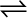7.8 Reaction between N2 and O2– takes place as follows:

2N2 (g) + O2 (g)2N2O (g)

If a mixture of 0.482 mol N2 and 0.933 mol of O2 is placed in a 10 L reaction vessel and allowed to form N2O at a temperature for which

Kc= 2.0 × 10–37, determine the composition of the equilibrium mixture.

Let the concentration of ${\mathrm{N}}_{2}\mathrm{O}$ at equilibrium be x.
The given reaction is :
Therefore, at equilibrium, in the 10L vessel:

The value of equilibrium constant i.e. ${\mathrm{K}}_{\mathrm{c}}=2.0×{10}^{-37}$ is very small. Therefore, the amount of  reacted is also very small. Thus, x can be neglected from the expressions of molar concentrations of . Then,